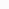# How to validate a credit card number?

All you know what information contains in your NIC number. But do you know what information contains in the Credit Card Number? Here are some useful details.

## Card Length

Typically, credit card numbers are all numeric and the length of the credit card number is between 12 digits to 19 digits.

• 14, 15, 16 digits – Diners Club
• 15 digits – American Express
• 13, 16 digits – Visa
• 16 digits – MasterCard

## Containing Information### 1 – Major Industry Identifier (MII)

The first digit of the credit card number is the Major Industry Identifier (MII). It designates the category of the entry which issued the card.

• 1 and 2 – Airlines
• 3 – Travel
• 4 and 5 – Banking and Financial
• 6 – Merchandising and Banking/Financial
• 7 – Petroleum
• 8 – Healthcare, Telecommunications
• 9 – National Assignment

### 2 – Issuer Identification Number

The first 6 digits are the Issuer Identification Number. It will identify the institution that issued the card. Following are some of the major IINs.

• Amex – 34xxxx, 37xxxx
• Visa – 4xxxxxx
• MasterCard – 51xxxx – 55xxxx
• Discover – 6011xx, 644xxx, 65xxxx

### 3 – Account Number

Taking away the 6 identifier digits and the last digits, remaining digits are the person’s account number (7th and following excluding last digits)

### 4 – Check digits

Last digit is known as check digits or checksum. It is used to validate the credit card number using Luhn algorithm (Mod 10 algorithm).

http://en.wikipedia.org/wiki/Bank_card_number
http://en.wikipedia.org/wiki/List_of_Issuer_Identification_Numbers

## Luhn algorithm (Mod 10)

The Luhn algorithm or Luhn formula, also known as the “modulus 10” or “mod 10” algorithm, is a simple checksum formula used to validate a variety of identification numbers, such as credit card numbers, IMEI numbers, National Provider Identifier numbers in US and Canadian Social Insurance Numbers. It was created by IBM scientist Hans Peter Luhn. (http://en.wikipedia.org/wiki/Luhn_algorithm)

When you implementing the ecommerce application, it is good practice to validate credit card number before send it to the bank validation. This saves a lot of time and money by avoiding a trip to the bank.

Here are the Luhn steps which can used to validate the credit card number.

### 4 0 1 2 8 8 8 8 8 8 8 8 1 8 8 1

1. Starting with the check digit double the value of every other digit (right to left every 2nd digit)2. If doubling of a number results in a two digits number, add up the digits to get a single digit number. This will results in eight single digit numbers3. Now add the un-doubled digits to the odd places4. Add up all the digits in this numberIf the final sum is divisible by 10, then the credit card number is valid. If it is not divisible by 10, the number is invalid.

Here is the code sample that I used to do the mod10 validation

```public static bool Mod10Check(string creditCardNumber)
{
//// check whether input string is null or empty
if (string.IsNullOrEmpty(creditCardNumber))
{
return false;
}

//// 1. Starting with the check digit double the value of every other digit
//// 2. If doubling of a number results in a two digits number, add up the digits to get a single digit number. This will results in eight single digit numbers
//// 3. Get the sum of the digits
int sumOfDigits = creditCardNumber.Where((e) => e >= '0' && e <= '9')
.Reverse()
.Select((e, i) => ((int)e - 48) * (i % 2 == 0 ? 1 : 2))
.Sum((e) => e / 10 + e % 10);
//// If the final sum is divisible by 10, then the credit card number is valid. If it is not divisible by 10, the number is invalid.
return sumOfDigits % 10 == 0;
}

```

The original article was modified according to the comments made by Code Project super users.